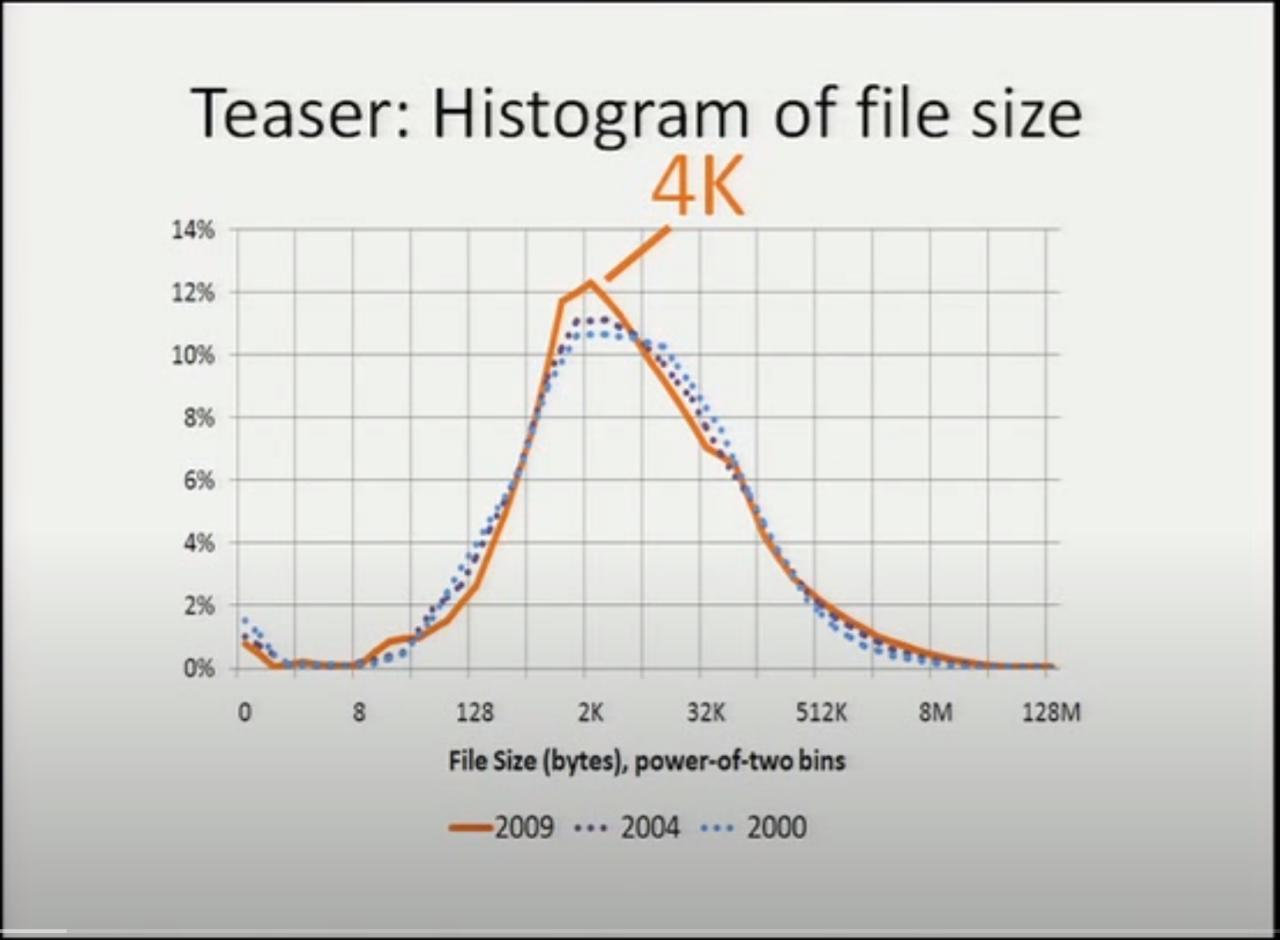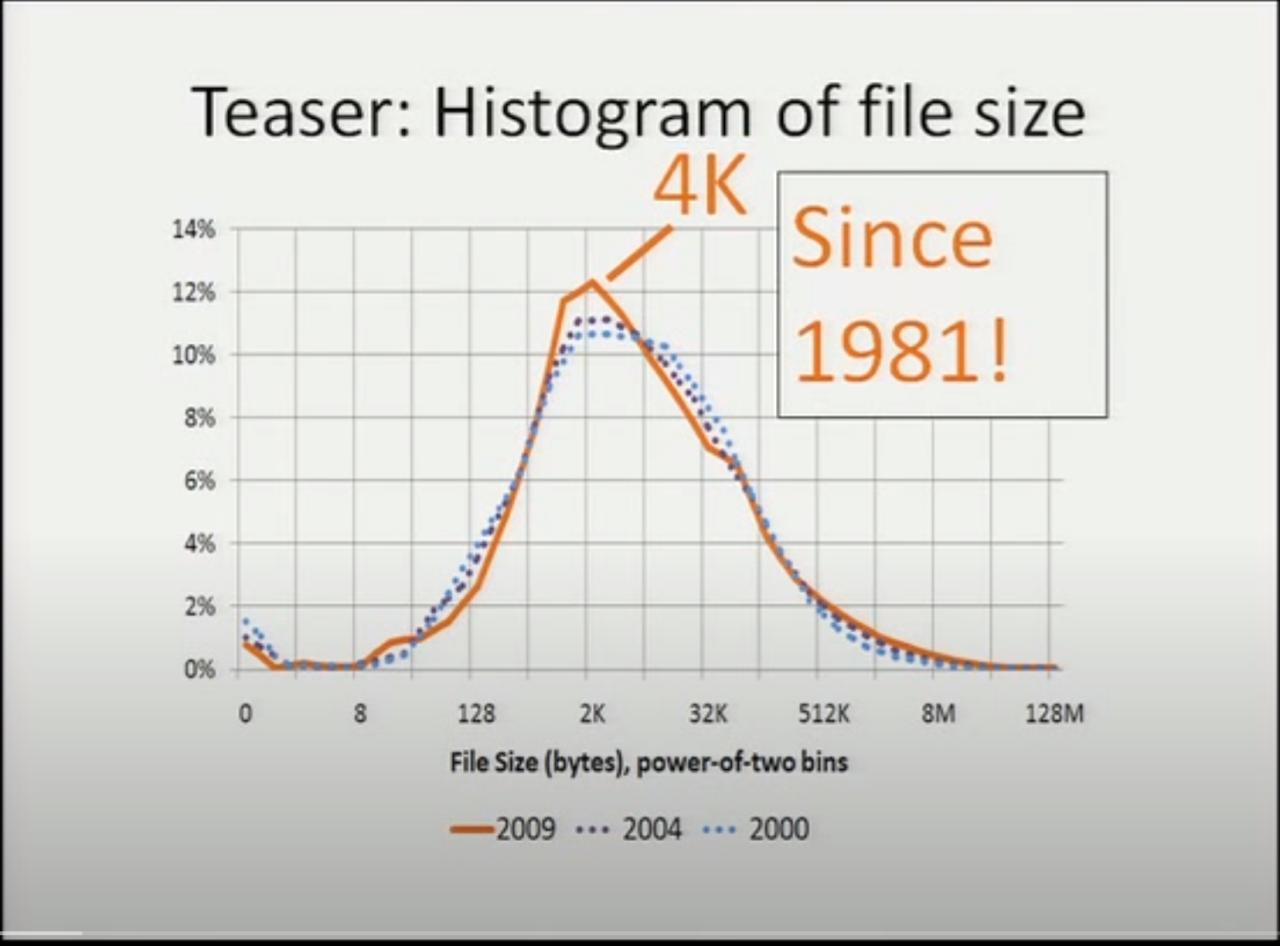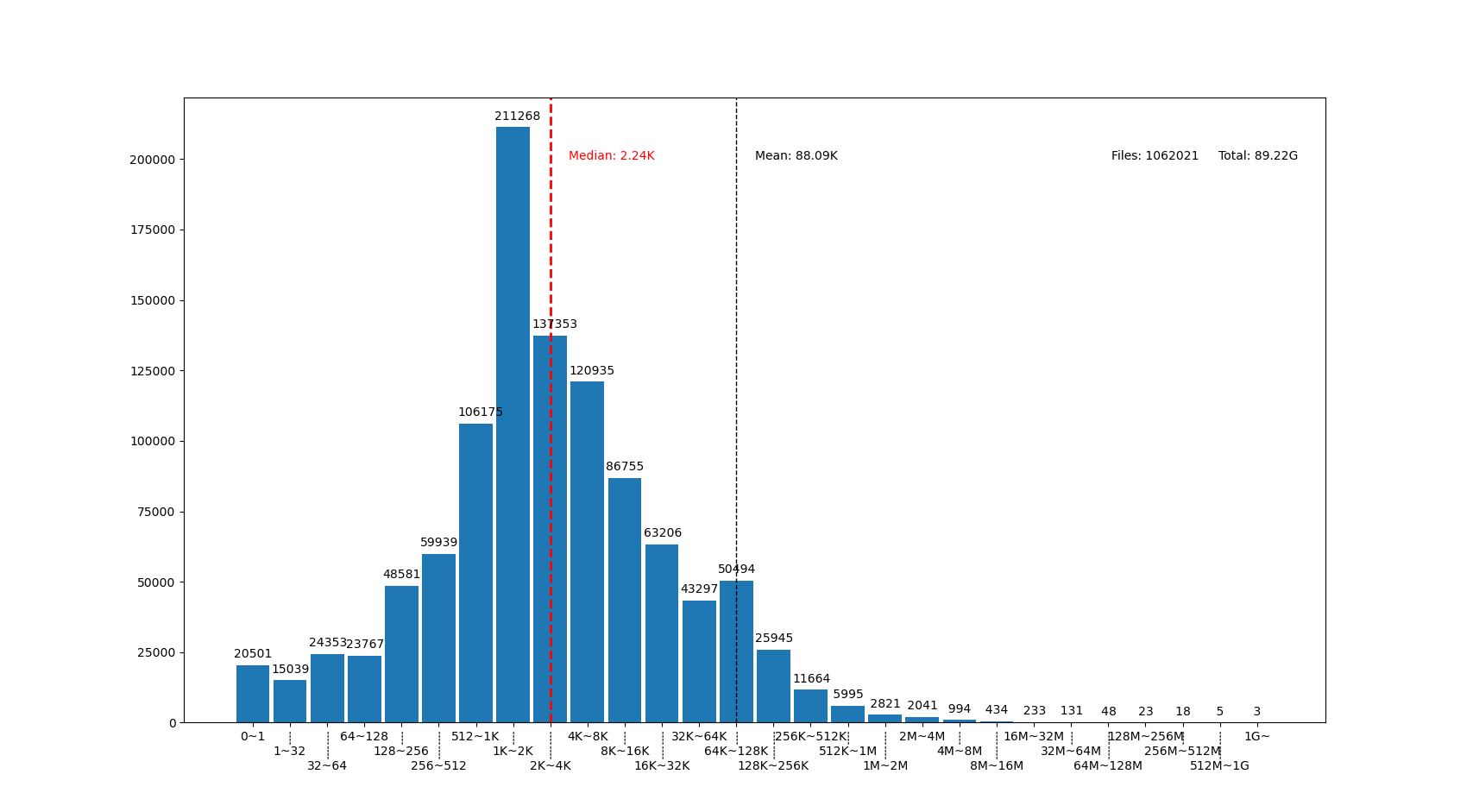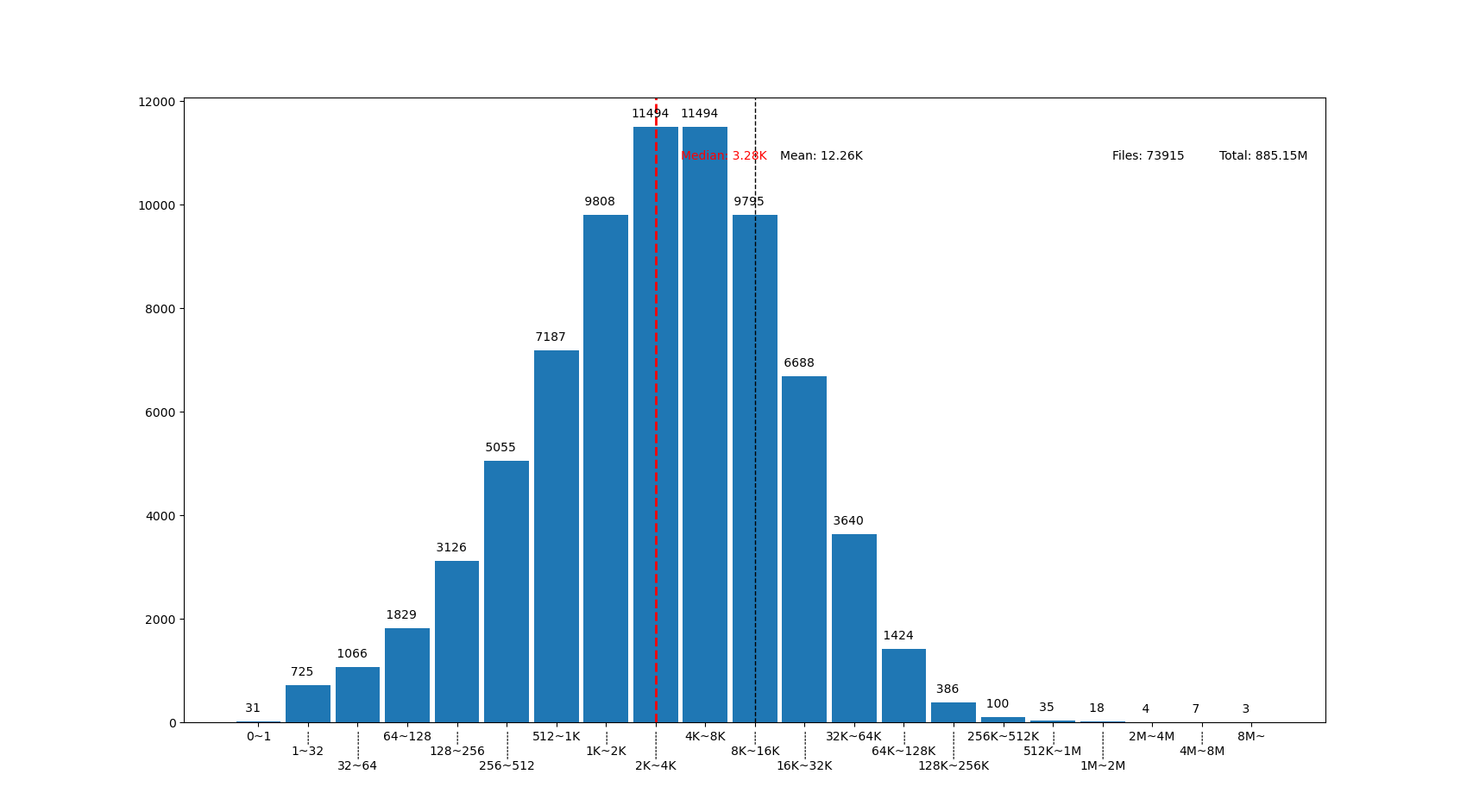# 系統中的大多數文件有多大？

## 你覺得，你的系統中大多數文件大概有多大？

• 我收藏了好多照片，每個有 2~5MiB 吧。
• 我下載了好多漫畫，每個 100KiB 左右，這些大概佔了不少比例。
• 我還收藏了不少動畫電影電視劇，雖然這些文件總數量可能不多？
• 我下載了 Linux 的源碼，那裏面每個 C 代碼文件都幾千行，每行 100 字寬，平均也得有 30KiB 吧，有幾萬個源碼文件呢，佔比應該挺大的……

• 統計學上說，大量獨立隨機事件的累積概率滿足正態分佈（常態分佈）曲線。假設我們把某個特定文件的大小增長 1字節看作是一次獨立隨機事件，那麼文件大小在文件系統中應該是滿足正態分佈的？
• 正態分佈的前提下，平均數接近中位數，文件系統的已佔用大小除以文件數量大概就是大部分文件的大小了吧。
• 根據我現在文件系統的佔用大小和文件數量，平均數大概是 500KiB 左右？
• 雖然我還存了幾個非常大，上 GiB 的文件，但是看起來似乎也有很多很多非常小的文件， 平均一下的話應該會把平均數拉大，大於中位數吧。那麼中位數應該在 100KiB 這樣的量級附近？

「我有個倉庫盤要存很多下載到的漫畫，每個漫畫都是一個文件夾裏面一堆 小 JPG ，每個就幾十 KiB 。網上看到的說法是 XFS 對 小文件 的性能不那麼好，我是不是該換 EXT4 ？我還想在 Windows 上能讀寫，是不是 ExFAT 這種簡單的文件系統更合適一點？」

「軟件源的鏡像服務器需要存的都是些 小文件 吧，大多數軟件包壓縮後也就是幾個 KiB 到幾個 MiB 的量級，這種需求是不是適合用對 小文件 優化比較好的文件系統？」

「我的程序需要分析的數據是大量幾百K的 小文件 ，該怎麼存合適呢，直接用文件系統還是應該上數據庫？ 我還想多線程併發分析，是不是 SQL 數據庫的併發能力強一些？又或者 MongoDB 的 GridFS 看起來似乎能結合文件系統和數據庫的特點，選它應該還不錯？」

## 統計實際系統中文件大小的學術研究…… the median file size is 4k. It was 4k the other two years of the study. We've actually gone back through the literature. It turns out it's 4k in every study going back to the last 30 years. So this is great news. We can finally compete with physicists: we have our own fundamental constant of the universe, it's a medium file size ……

## 不信？你可以測一下自己的文件系統

```find /home -type f -printf "%s %p\n" > myhome.txt
```

```#!/usr/bin/python3
import argparse
import matplotlib.pyplot as plt
import numpy as np
import sys
from math import *
from bisect import bisect_left

def numfmt(s):
marks = "KMGTP"
m = 0
f = type(s) is float
while s >= 1024 and m < len(marks):
if f:
s /= 1024.0
else:
s //=1024
m += 1
if f:
return f"{s:.2f}{marks[m-1:m]}"
else:
return f"{s}{marks[m-1:m]}"

if __name__ == '__main__':
parser = argparse.ArgumentParser(
prog = "filesizehistogram",
description = """
can use "-" as input filename, indicate input is taken from stdin.
otherwise input file should be a result of "find -printf \'%s %p\\n\'"
"""
)
parser.add_argument('-o', '--output', help="output filename, will recognize common extensions by matplot")
args = parser.parse_args()

filenames = [x if x != '-' else '/dev/stdin' for x in args.input]
data=np.array([int(x.split(' ')) for fn in filenames for x in open(fn)])
mindatalog2 = 5 # cut from 32
maxdatalog2 = min(ceil(log2(data.max())), 31) # cut at 1G and above
# bins [0, 1, 32, 64, 128, 256, ... , 1G, 2G] , last bin is open range
bins=[0,1,] + [2**x for x in range(mindatalog2, maxdatalog2 + 1)]

median = float(np.median(data))
mean = float(data.mean())
bmedian = bisect_left(bins, median) - 1
bmean = bisect_left(bins, mean) - 1
files = len(data)
total = data.sum()

hist, bin_edges = np.histogram(data,bins)
fig,ax = plt.subplots(figsize=(20,8))
ax.bar(range(len(hist)), hist, width=0.9)
ax.set_xticks([i for i in range(len(hist))])
tickbar = "┊\n"
ax.set_xticklabels([f'{tickbar*(i%3)}{numfmt(bins[i])}~{numfmt(bins[i+1])}' for i in range(len(hist)-1)] +
[f"{numfmt(bins[len(hist)-1])}~"])

ax.axvline(bmean, color='k', linestyle='dashed', linewidth=1)
ax.axvline(bmedian, color='r', linestyle='dashed', linewidth=2)
min_ylim, max_ylim = plt.ylim()
min_xlim, max_xlim = plt.xlim()
ax.text(bmean + 0.5   , max_ylim * 0.9, f'Mean: {numfmt(mean)}')
ax.text(bmedian + 0.5 , max_ylim * 0.9, f'Median: {numfmt(median)}', color='r')
ax.text(max_xlim * 0.8, max_ylim * 0.9, f'Files: {files}')
ax.text(max_xlim * 0.9, max_ylim * 0.9, f'Total: {numfmt(float(total))}')

for i in range(len(hist)):
ax.text(i - 0.5, hist[i] + files / 400, f"{hist[i]:5}") # label on top of every bar, uplefted a little

if args.output:
plt.savefig(args.output)
else:
plt.show()
```linux 代碼樹的文件大部分比我猜的 30K 要小呢，主要在 1K~16K ，中位數 3.28K 。而且意外得在代碼樹裏有好幾個 0 大小的文件，看了幾個文件路徑確認了一下，它們的確是 0 大小的頭文件，並不是我的文件系統丟了文件內容。